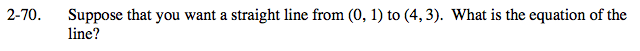### Home > PC > Chapter 2 > Lesson 2.3.1 > Problem2-70

2-70.

Suppose that you want a straight line from (0, 1) to (4, 3). What is the equation of the line? Homework Help ✎What is the other piece of information you need for the equation of a straight line?

Slope